# Create and use a scatter plot matrix

A scatter plot matrix is a grid of several scatter plots of up to five numeric variables. The matrix includes individual scatter plots for every combination of variables.

A scatter plot matrix can answer questions about your data, such as: What are the relationships between several variables?

## Examples

A public works department has noticed an increase in leaks on water mains. The department wants to know whether the length of pipes and the average diameter have an effect on the number of leaks. A scatter plot matrix can be created to determine the relationships between the length and diameter of pipes and the number of leaks.

## Create a scatter plot matrix

To create a scatter plot matrix, complete the following steps:

1. Select three to five number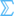or rate/ratio fields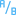.
##### Note:

You can search for fields using the search bar in the data pane.

2. Create the scatter plot matrix using the following steps:
1. Drag the selected fields to a new card.
2. Drop the selected fields on the Scatter Plot Matrix drop zone.
##### Tip:

You can also create charts using the Chart menu above the data pane or the Visualization type button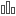on an existing card. For the Chart menu, only charts that are compatible with your data selection will be enabled. For the Visualization type menu, only compatible visualizations (including maps, charts, or tables) will be displayed.

##### Note:

When you drag three to five number fields onto your page, the Chart drop zone is replaced with the Scatter Plot Matrix drop zone. The reason for the change is because a scatter plot matrix is the only chart type that can be created using three number fields.

## Usage notes

Scatter plot matrices are symbolized using single symbols if a Color by variable is not used, or by unique values if there is a Color by variable assigned. The default single symbol has no fill to make it easier to read plots with large numbers of points.

The Legend button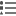opens the Layer options pane. The Layer options pane contains the following functions:

• The Legend tabis available when a Color by variable is applied to the x-axis on the chart. The pop out legend button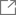displays the legend as a separate card on your page. The legend can be used to make selections on the chart. To change the color associated with a value, click the symbol and choose a color from the palette or enter a hex value.
• The Style tab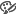changes the symbol color (single symbol only) and the outline color on the chart.

Use the Flip Fields button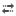to switch the variables on the x- and y-axes.

Use the Visualization type buttonto switch directly between a scatter plot matrix and a summary table.

The R2 values for each plot are displayed in a corresponding grid in the empty space of the card. The R2 value can be used to analyze the strength of the relationship between the variables in each scatter plot, with R2 values closer to 1 indicating a stronger linear relationship.

##### Note:

R2 values are not supported for certain remote feature layers. If you need the R2 value for an unsupported remote feature layer, you can drag the individual charts to the Scatter Plot drop zone and use the Chart Statistics button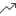to create a trend line or copy the dataset to your workbook and create a scatter plot matrix with the copy.

Create a full scatter plot from the matrix by selecting a plot and dragging it to create a new card.

## Regression analysis

Scatter plot matrices are an important part of regression analysis. Multiple scatter plot matrices are required for the exploratory analysis of your regression model to test the assumptions of Ordinary Least Squares (OLS).

A scatter plot matrix can be created for the dependent and exploratory variables by clicking the Visualize button on the Create Regression Model pane.